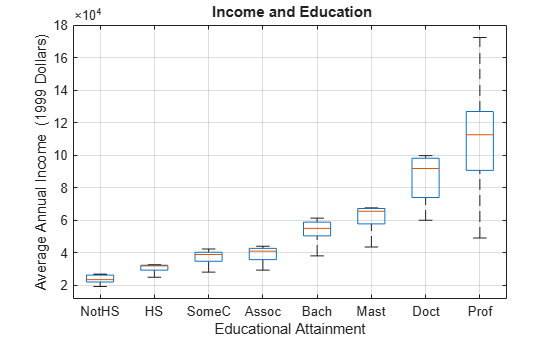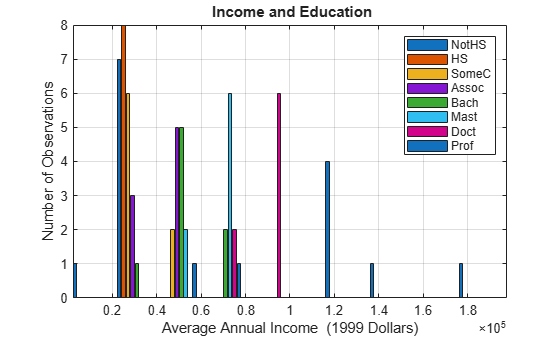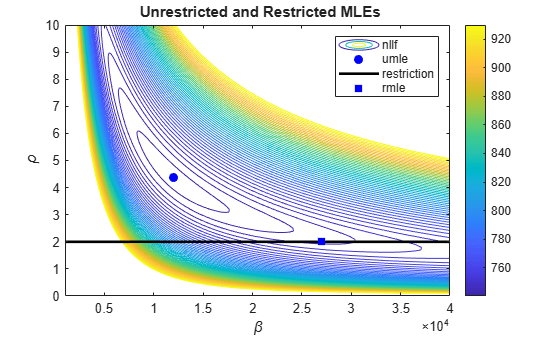Documentation

Classical Model Misspecification Tests

This example shows the use of the likelihood ratio, Wald, and Lagrange multiplier tests. These tests are useful in the evaluation and assessment of model restrictions and, ultimately, the selection of a model that balances the often competitive goals of adequacy and simplicity.

Introduction

Econometric models are a balance. On the one hand, they must be sufficiently detailed to account for relevant economic factors and their influence on observed data patterns. On the other hand, they must avoid unnecessary complexities that lead to computational challenges, over-fitting, or problems with interpretation. Working models are often developed by considering a sequence of nested specifications, in which larger, theoretical models are examined for simplifying restrictions on the parameters. If the parameters are estimated by maximum likelihood, three classical tests are typically used to assess the adequacy of the restricted models. They are the likelihood ratio test, the Wald test, and the Lagrange multiplier test.

The loglikelihood of model parameters $\theta$, given data $d$, is denoted $L$($\theta$ | $d$). With no restrictions on the model, $L$ is optimized at the maximum likelihood estimate (MLE) $\underset{}{\overset{ˆ}{\theta }}$. With restrictions of the form $r$($\theta$) $=0$, $L$ is optimized at $\underset{}{\overset{\sim }{\theta }}$ with a generally reduced loglikelihood of describing the data. The classical tests evaluate the statistical significance of model restrictions using information obtained from these optimizations. The framework is very general; it encompasses both linear and nonlinear models, and both linear and nonlinear restrictions. In particular, it extends the familiar framework of t and F tests for linear models.

Each test uses the geometry of the loglikelihood surface to evaluate the significance of model restrictions in a different way:

• The likelihood ratio test considers the difference in loglikelihoods at $\underset{}{\overset{ˆ}{\theta }}$ and $\underset{}{\overset{\sim }{\theta }}$. If the restrictions are insignificant, this difference should be near zero.

• The Wald test considers the value of $r$ at $\underset{}{\overset{ˆ}{\theta }}$. If the restrictions are insignificant, this value should be near the value of $r$ at $\underset{}{\overset{\sim }{\theta }}$, which is zero.

• The Lagrange multiplier test considers the gradient, or score, of $L$ at $\underset{}{\overset{\sim }{\theta }}$. If the restrictions are insignificant, this vector should be near the score at $\underset{}{\overset{ˆ}{\theta }}$, which is zero.

The likelihood ratio test evaluates the difference in loglikelihoods directly. The Wald and Lagrange multiplier tests do so indirectly, with the idea that insignificant changes in the evaluated quantities can be identified with insignificant changes in the parameters. This identification depends on the curvature of the loglikelihood surface in the neighborhood of the MLE. As a result, the Wald and Lagrange multiplier tests include an estimate of parameter covariance in the formulation of the test statistic.

Econometrics Toolbox™ software implements the likelihood ratio, Wald, and Lagrange multiplier tests in the functions lratiotest, waldtest, and lmtest, respectively.

Data and Models

Consider the following data from the U.S. Census Bureau, giving average annual earnings by educational attainment level:

numLevels = 8;
X = 100*repmat(1:numLevels,numLevels,1); % Levels: 100, 200, ..., 800
x = X(:);       % Education
y = Data(:);    % Income
n = length(y);  % Sample size

levelNames = DataTable.Properties.VariableNames;
boxplot(Data,'labels',levelNames)
grid on

xlabel('Educational Attainment')
ylabel('Average Annual Income  (1999 Dollars)')
title('{\bf Income and Education}')The income distributions in the data are conditional on the educational attainment level x. This pattern is also evident in a histogram of the data, which shows the small sample size:

figure

edges = [0:0.2:2]*1e5;
centers =[0.1:0.2:1.9]*1e5;
BinCounts = zeros(length(edges)-1,numLevels);

for j = 1:numLevels
BinCounts(:,j) = histcounts(Data(:,j),edges);
end;

h = bar(centers,BinCounts);
axis tight
grid on
legend(h,levelNames)
xlabel('Average Annual Income  (1999 Dollars)')
ylabel('Number of Observations')
title('{\bf Income and Education}')A common model for this type of data is the gamma distribution, with conditional density

$f\left({y}_{i}|{x}_{i},\beta ,\rho \right)=\frac{{\beta }_{i}^{\rho }}{\Gamma \left(\rho \right)}{y}^{\rho -1}{e}^{-{y}_{i}{\beta }_{i}},$

where

${\beta }_{i}=1/\left(\beta +{x}_{i}\right)$

and

$i=1,...,n.$

Gamma distributions are sums of $\rho$ exponential distributions, and so admit natural restrictions on the value of $\rho$. The exponential distribution, with $\rho$ equal to 1, is monotonically decreasing and too simple to describe the unimodal distributions in the data. For the purposes of illustration, we will maintain a restricted model that is the sum of two exponential distributions, obtained by imposing the restriction

$r\left(\beta ,\rho \right)=\rho -2=0.$

This null model will be tested against the unrestricted alternative represented by the general gamma distribution.

The loglikelihood function of the conditional gamma density, and its derivatives, are found analytically:

$L\left(\beta ,\rho |x\right)=\rho \sum _{i=1}^{n}\mathrm{ln}{\beta }_{i}-n\mathrm{ln}\Gamma \left(\rho \right)+\left(\rho -1\right)\sum _{i=1}^{n}\mathrm{ln}{y}_{i}-\sum _{i=1}^{n}{y}_{i}{\beta }_{i}$

$\frac{\partial L}{\partial \beta }=-\rho \sum _{i=1}^{n}{\beta }_{i}+\sum _{i=1}^{n}{y}_{i}{\beta }_{i}^{2}$

$\frac{\partial L}{\partial \rho }=\sum _{i=1}^{n}\mathrm{ln}{\beta }_{i}-n\Psi \left(\rho \right)+\sum _{i=1}^{n}\mathrm{ln}{y}_{i}$

$\frac{{\partial }^{2}L}{\partial {\beta }^{2}}=\rho \sum _{i=1}^{n}{\beta }_{i}^{2}-2\sum _{i=1}^{n}{y}_{i}{\beta }_{i}^{3}$

$\frac{{\partial }^{2}L}{\partial {\rho }^{2}}=-n{\Psi }^{\prime }\left(\rho \right)$

$\frac{{\partial }^{2}L}{\partial \beta \partial \rho }=-\sum _{i=1}^{n}{\beta }_{i}$

where $\Psi$ is the digamma function, the derivative of $\mathrm{ln}\Gamma$.

The loglikelihood function is used to find MLEs for the restricted and unrestricted models. The derivatives are used to construct gradients and parameter covariance estimates for the Wald and Lagrange multiplier tests.

Maximum Likelihood Estimation

Since optimizers in MATLAB® and Optimization Toolbox™ software find minima, we maximize the loglikelihood by minimizing the negative loglikelihood function. Using the $L$ found above, we code the negative loglikelihood function with parameter vector p = [beta;rho]:

nLLF = @(p)sum(p(2)*(log(p(1)+x))+gammaln(p(2))-(p(2)-1)*log(y)+y./(p(1)+x));

We use the function fmincon to compute the restricted parameter estimates at $\rho$ = 2. The lower bound on $\beta$ assures that the logarithm in nLLF is evaluated at positive arguments:

options = optimoptions(@fmincon,'Algorithm','active-set','TolFun',1e-10,'Display','off');

rp0 = [1 1];        % Initial values
rlb = [-min(x) 2];  % Lower bounds
rub = [Inf 2];      % Upper bounds
[rmle,rnLL] = fmincon(nLLF,rp0,[],[],[],[],rlb,rub,[],options);
rbeta = rmle(1);    % Restricted beta estimate
rrho = rmle(2);     % Restricted rho estimate
rLL = -rnLL;        % Restricted loglikelihood

Unrestricted parameter estimates are computed in a similar manner, starting from initial values given by the restricted estimates:

up0 = [rbeta rrho]; % Initial values
ulb = [-min(x) 0];  % Lower bounds
uub = [Inf Inf];    % Upper bounds
[umle,unLL] = fmincon(nLLF,up0,[],[],[],[],ulb,uub,[],options);
ubeta = umle(1);    % Unrestricted beta estimate
urho = umle(2);     % Unrestricted rho estimate
uLL = -unLL;        % Unrestricted loglikelihood

We display the MLEs on a logarithmic contour plot of the negative loglikelihood surface:

betas = 1e3:1e2:4e4;
rhos = 0:0.1:10;
[BETAS,RHOS] = meshgrid(betas,rhos);
NLL = zeros(size(BETAS));
for i = 1:numel(NLL)
NLL(i) = nLLF([BETAS(i),RHOS(i)]);
end
L = log10(unLL);
v = logspace(L-0.1,L+0.1,100);

contour(BETAS,RHOS,NLL,v) % Negative loglikelihood surface
colorbar
hold on
plot(ubeta,urho,'bo','MarkerFaceColor','b')     % Unrestricted MLE
line([1e3 4e4],[2 2],'Color','k','LineWidth',2) % Restriction
plot(rbeta,rrho,'bs','MarkerFaceColor','b')     % Restricted MLE

legend('nllf','umle','restriction','rmle')
xlabel('\beta')
ylabel('\rho')
title('{\bf Unrestricted and Restricted MLEs}')Covariance Estimators

The intuitive relationship between the curvature of the loglikelihood surface and the variance/covariance of the parameter estimates is formalized by the information matrix equality, which identifies the negative expected value of the Hessian with the Fisher information matrix. The second derivatives in the Hessian express loglikelihood concavities. The Fisher information matrix expresses parameter variance; its inverse is the asymptotic covariance matrix.

Covariance estimators required by the Wald and Lagrange multiplier tests are computed in a variety of ways. One approach is to use the outer product of gradients (OPG), which requires only first derivatives of the loglikelihood. While popular for its relative simplicity, the OPG estimator can be unreliable, especially with small samples. Another, often preferable, estimator is the inverse of the negative expected Hessian. By the information matrix equality, this estimator is the asymptotic covariance, appropriate for large samples. If analytic expectations are difficult to compute, the expected Hessian can be replaced by the Hessian evaluated at the parameter estimates, the so-called "observed" Fisher information.

We compute each of the three estimators, using the derivatives of $L$ found earlier. Conditional expectations in the Hessian are found using

$E\left[g\left(X\right)Y|X\right]=g\left(X\right)E\left[Y|X\right].$

We evaluate the estimators at the unrestricted parameter estimates, for the Wald test, and then at the restricted parameter estimates, for the Lagrange multiplier test.

Different scales for the $\beta$ and $\rho$ parameters are reflected in the relative sizes of the variances. The small sample size is reflected in the differences among the estimators. We increase the precision of the displays to show these differences:

format long

Evaluated at the unrestricted parameter estimates, the estimators are:

% OPG estimator:

UG = [-urho./(ubeta+x)+y.*(ubeta+x).^(-2),-log(ubeta+x)-psi(urho)+log(y)];
Uscore = sum(UG)';
UEstCov1 = inv(UG'*UG) %#ok
UEstCov1 = 2×2
106 ×

6.163712448531872  -0.002335592524740
-0.002335592524740   0.000000949846859

% Hessian estimator (observed information):

UDPsi = (psi(urho+0.0001)-psi(urho-0.0001))/(0.0002); % Digamma derivative
UH = [sum(urho./(ubeta+x).^2)-2*sum(y./(ubeta+x).^3),-sum(1./(ubeta+x)); ...
-sum(1./(ubeta+x)),-n*UDPsi];
UEstCov2 = -inv(UH) %#ok
UEstCov2 = 2×2
106 ×

5.914328160244167  -0.001864358576657
-0.001864358576657   0.000000648727099

% Expected Hessian estimator (expected information):

UEH = [-sum(urho./((ubeta+x).^2)), -sum(1./(ubeta+x)); ...
-sum(1./(ubeta+x)),-n*UDPsi];
UEstCov3 = -inv(UEH) %#ok
UEstCov3 = 2×2
106 ×

4.993552448927904  -0.001574104798365
-0.001574104798365   0.000000557231141

Evaluated at the restricted parameter estimates, the estimators are:

% OPG estimator:

RG = [-rrho./(rbeta+x)+y.*(rbeta+x).^(-2),-log(rbeta+x)-psi(rrho)+log(y)];
Rscore = sum(RG)';
REstCov1 = inv(RG'*RG) %#ok
REstCov1 = 2×2
107 ×

6.614319529708872  -0.000476569582451
-0.000476569582451   0.000000040110436

% Hessian estimator (observed information):

RDPsi = (psi(rrho+0.0001)-psi(rrho-0.0001))/(0.0002); % Digamma derivative
RH = [sum(rrho./(rbeta+x).^2)-2*sum(y./(rbeta+x).^3),-sum(1./(rbeta+x)); ...
-sum(1./(rbeta+x)),-n*RDPsi];
REstCov2 = -inv(RH) %#ok
REstCov2 = 2×2
107 ×

2.708403012378076  -0.000153134581292
-0.000153134581292   0.000000011081041

% Expected Hessian estimator (expected information):

REH = [-sum(rrho./((rbeta+x).^2)),-sum(1./(rbeta+x)); ...
-sum(1./(rbeta+x)),-n*RDPsi];
REstCov3 = -inv(REH) %#ok
REstCov3 = 2×2
107 ×

2.613661653125537  -0.000147777853245
-0.000147777853245   0.000000010778169

format short

The Likelihood Ratio Test

The likelihood ratio test, which evaluates the statistical significance of the difference in loglikelihoods at the unrestricted and restricted parameter estimates, is generally considered to be the most reliable of the three classical tests. Its main disadvantage is that it requires estimation of both models. This may be an issue if either the unrestricted model or the restrictions are nonlinear, making significant demands on the necessary optimizations.

Once the required loglikelihoods have been obtained through maximum likelihood estimation, use lratiotest to run the likelihood ratio test:

dof = 1; % Number of restrictions
[LRh,LRp,LRstat,cV] = lratiotest(uLL,rLL,dof) %#ok
LRh = logical
1

LRp = 7.9882e-05
LRstat = 15.5611
cV = 3.8415

The test rejects the restricted model (LRh = 1), with a p-value (LRp = 7.9882e-005) well below the default significance level (alpha = 0.05), and a test statistic (LRstat = 15.5611) well above the critical value (cV = 3.8415).

Like the Wald and Lagrange multiplier tests, the likelihood ratio test is asymptotic; the test statistic is evaluated with a limiting distribution obtained by letting the sample size tend to infinity. The same chi-square distribution, with degree of freedom dof, is used to evaluate the individual test statistics of each of the three tests, with the same critical value cV. Consequences for drawing inferences from small samples should be apparent, and this is one of the reasons why the three tests are often used together, as checks against one another.

The Wald Test

The Wald test is appropriate in situations where the restrictions impose significant demands on parameter estimation, as in the case of multiple nonlinear constraints. The Wald test has the advantage that it requires only the unrestricted parameter estimate. Its main disadvantage is that, unlike the likelihood ratio test, it also requires a reasonably accurate estimate of the parameter covariance.

To perform a Wald test, restrictions must be formulated as functions from p-dimensional parameter space to q-dimensional restriction space:

$r=\left(\begin{array}{c}{r}_{1}\left({\theta }_{1},\dots ,{\theta }_{p}\right)\\ ⋮\\ {r}_{q}\left({\theta }_{1},\dots ,{\theta }_{p}\right)\end{array}\right)$

with Jacobian

$R=\left(\begin{array}{ccc}\frac{\partial {r}_{1}}{\partial {\theta }_{1}}& \dots & \frac{\partial {r}_{1}}{\partial {\theta }_{p}}\\ ⋮& \ddots & ⋮\\ \frac{\partial {r}_{q}}{\partial {\theta }_{1}}& \dots & \frac{\partial {r}_{q}}{\partial {\theta }_{p}}\end{array}\right).$

For the gamma distribution under consideration, the single restriction

$r\left(\beta ,\rho \right)=\rho -2$

maps 2-dimensional parameter space to 1-dimensional restriction space with Jacobian [0 1].

Use waldtest to run the Wald test with each of the unrestricted covariance estimates computed previously. The number of restrictions dof is the length of the input vector r, so it does not have to be input explicitly as for lratiotest or lmtest:

r = urho-2; % Restriction vector
R = [0 1];  % Jacobian
restrictions = {r,r,r};
Jacobians = {R,R,R};
UEstCov = {UEstCov1,UEstCov2,UEstCov3};
[Wh,Wp,Wstat,cV] = waldtest(restrictions,Jacobians,UEstCov) %#ok
Wh = 1x3 logical array

1   1   1

Wp = 1×3

0.0144    0.0031    0.0014

Wstat = 1×3

5.9877    8.7671   10.2066

cV = 1×3

3.8415    3.8415    3.8415

The test rejects the restricted model with each of the covariance estimates.

Hypothesis tests in Econometrics Toolbox and Statistics Toolbox™ software operate at a default 5% significance level. The significance level can be changed with an optional input:

alpha = 0.01; % 1% significance level
[Wh2,Wp2,Wstat2,cV2] = waldtest(restrictions,Jacobians,UEstCov,alpha) %#ok
Wh2 = 1x3 logical array

0   1   1

Wp2 = 1×3

0.0144    0.0031    0.0014

Wstat2 = 1×3

5.9877    8.7671   10.2066

cV2 = 1×3

6.6349    6.6349    6.6349

The OPG estimator fails to reject the restricted model at the new significance level.

The Lagrange Multiplier Test

The Lagrange multiplier test is appropriate in situations where the unrestricted model imposes significant demands on parameter estimation, as in the case where the restricted model is linear but the unrestricted model is not. The Lagrange multiplier test has the advantage that it requires only the restricted parameter estimate. Its main disadvantage is that, like the Wald test, it also requires a reasonably accurate estimate of the parameter covariance.

Use lmtest to run the Lagrange multiplier test with each of the restricted covariance estimates computed previously:

scores = {Rscore,Rscore,Rscore};
REstCov = {REstCov1,REstCov2,REstCov3};
[LMh,LMp,LMstat,cV] = lmtest(scores,REstCov,dof) %#ok
LMh = 1x3 logical array

1   1   1

LMp = 1×3

0.0000    0.0024    0.0027

LMstat = 1×3

33.4617    9.2442    8.9916

cV = 1×3

3.8415    3.8415    3.8415

The test again rejects the restricted model with each of the covariance estimates at the default significance level. The reliability of the OPG estimator is called into question by the anomalously large value of the first test statistic.

Summary

The three classical model misspecification tests form a natural toolkit for econometricians. In the context of maximum likelihood estimation, they all attempt to make the same distinction, between an unrestricted and a restricted model in some hierarchy of progressively simpler descriptions of the data. Each test, however, comes with different requirements, and so may be useful in different modeling situations, depending on the computational demands. When used together, inferences can vary among the tests, especially with small samples. Users should consider the tests as only one component of a wider statistical and economic analysis.

References

 Davidson, R. and J.G. MacKinnon. Econometric Theory and Methods. Oxford, UK: Oxford University Press, 2004.

 Godfrey, L.G. Misspecification Tests in Econometrics. Cambridge, UK: Cambridge University Press, 1997.

 Greene, W.H. Econometric Analysis. Upper Saddle River, NJ: Pearson Prentice Hall, 2008.

 Hamilton, J.D. Time Series Analysis. Princeton, NJ: Princeton University Press, 1994.